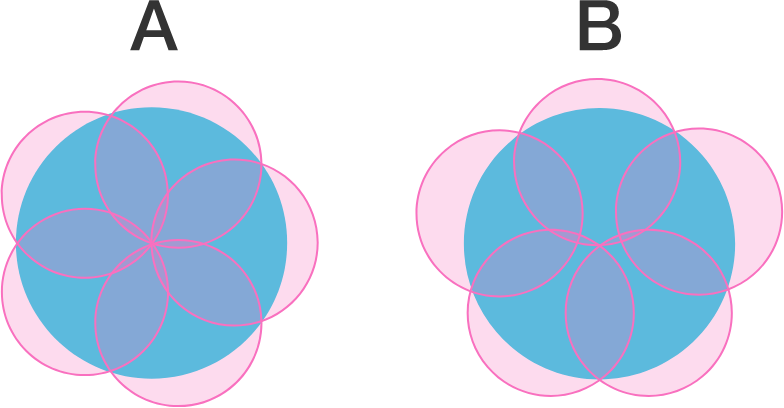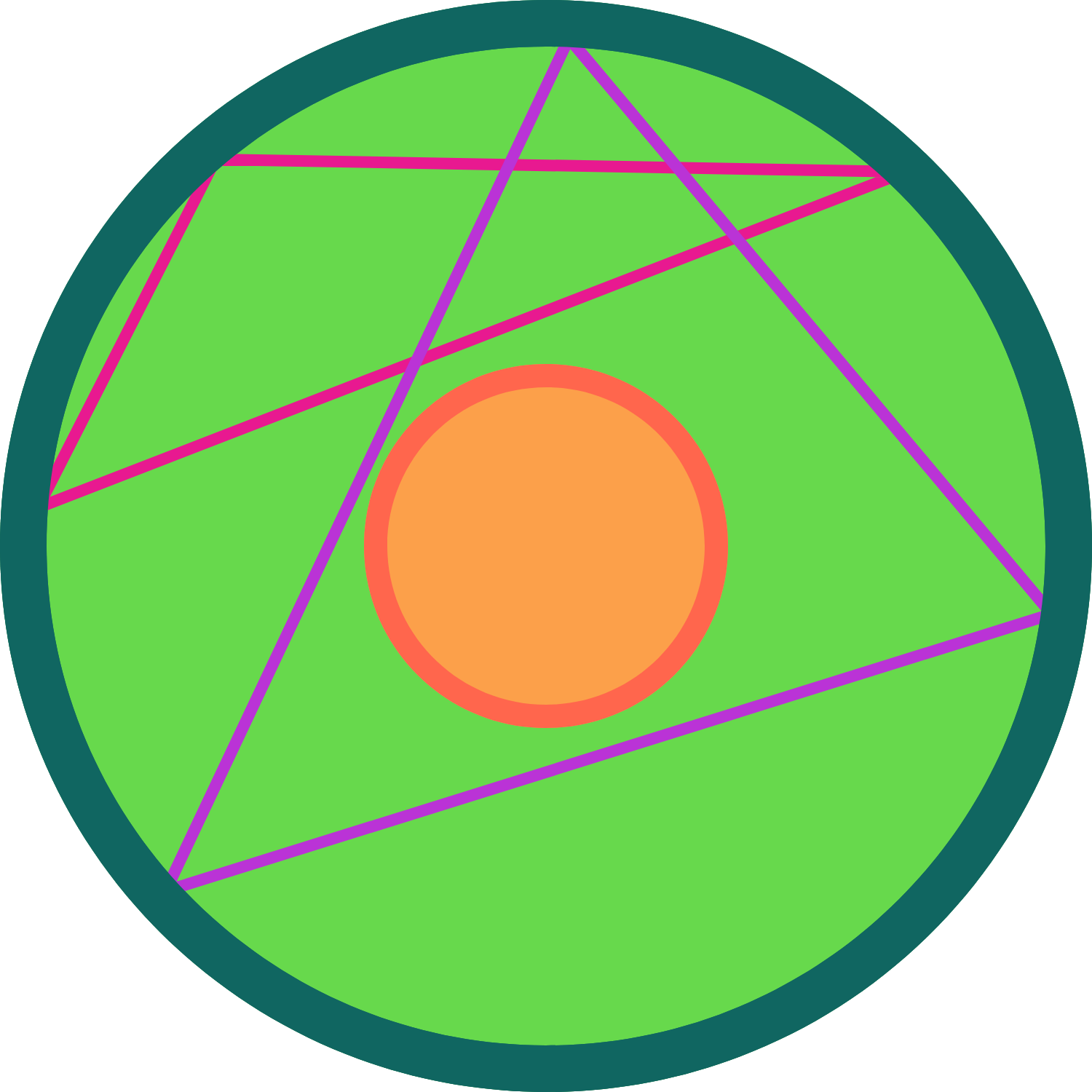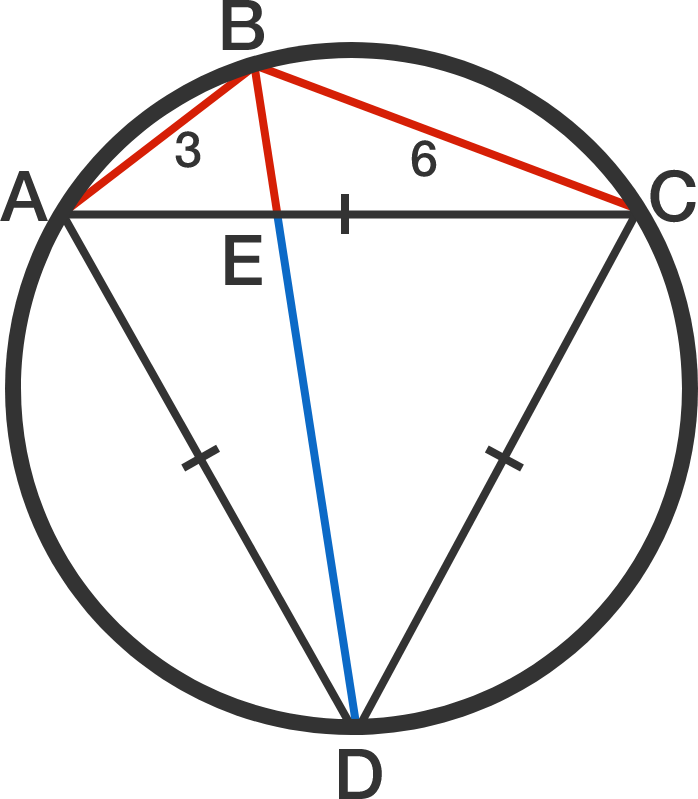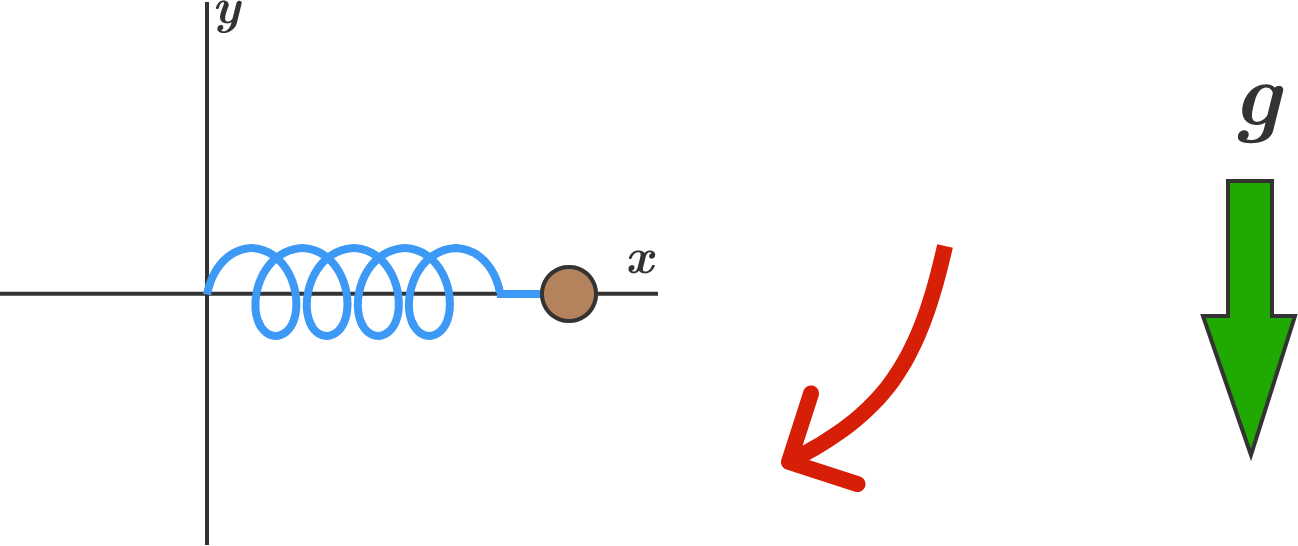# Problems of the Week

Contribute a problemYou go to a carnival and decide to play the Cover the Spot game.

The rules are simple: you must cover the largest circular spot possible using 5 identical circular disks. Which configuration can cover a larger spot?

A) All 5 disks pass through the center of the spot.
B) Exactly 3 disks pass through the center of the spot.Circles $L$ and $l$ share the same center. Circle $L$ has radius $3r$ and circle $l$ has radius $r.$ Points $A, B, C$ are chosen on the circumference of circle $L$ uniformly at random.

What is the probability that the centroid of $\triangle ABC$ lies in the interior of circle $l$?A cyclic quadrilateral $ABCD$ is constructed within a circle such that $AB = 3, BC = 6,$ and $\triangle ACD$ is equilateral, as shown to the right.

If $E$ is the intersection point of both diagonals of $ABCD$, what is the length of $ED,$ the blue line segment in the diagram?

$\frac{m^3-n^3}{m^2+n^2-mn}$

Find the sum of all prime numbers less than 900 that can be expressed as the above fraction where $m$ and $n$ are positive integers.In the $xy$-coordinate system, a $\SI{1}{\kilo\gram}$ mass is attached to one end of a massless ideal spring. The other end of the spring is fixed at the origin. The spring constant is $k = \SI[per-mode=symbol]{10}{\newton\per\meter}$, and the spring's unstretched length is $\SI{1}{\meter}$.

The mass is initially being held in the air horizontally at $(x,y) = (\SI{1}{\meter}, \SI{0}{\meter}),$ and then is released so that gravity pulls it downwards. How far is the mass from the origin right at the moment it first crosses the (vertical) $y$-axis?


Details and Assumptions:

• There is an ambient downward gravitational acceleration of $\SI[per-mode=symbol]{10}{\meter\per\second\squared}$.